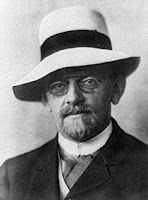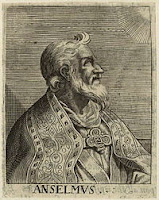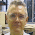## Thursday, June 7, 2018

### Gödel and realismKurt Gödel

Kurt Gödel (1906-1978) was one of the most important mathematicians of the 20th century. In 1931, when he was 25, he rose to fame with his mathematical proof that the attempt to build a complete axiomatic system, from which one can deduce all the arithmetic of natural numbers or any equivalent system, is doomed to failure.
His first incompleteness theorem says the following:
Every consistent formal system as powerful as elementary arithmetic is not complete (it contains true undecidable propositions).
Let us look at a simplified informal demonstration:
Let theorem G say the following: This theorem G cannot be proved from the axioms and rules of system S.
• If we assume that Theorem G is false, system S is inconsistent, since a false theorem can be proved from the axioms and rules of S.
• Then if S is consistent, G must be true, and therefore cannot be proved from the axioms of S.
Gödel’s theorem shows that every axiomatic formalization of arithmetic is either inconsistent (it allows false theorems to be proved), or incomplete (it contains true theorems that cannot be proved).David Hilbert
The German mathematician David Hilbert published a list of 23 outstanding pending problems of mathematics. In the second place of his list he posed the following: Prove that the axioms of arithmetic are consistent.
Gödel's second incompleteness theorem says this:
Given a theory T, generated from basic arithmetic axioms and formal rules of deduction, if T contains the proof of its own consistency, then T is inconsistent.
In other words, Gödel’s second theorem proves that the second Hilbert problem has no solution.
What did Kurt Gödel think about the dilemma between realism and anti-realism? To verify that Gödel was a Platonic realist, it is enough to look at this paragraph he wrote in a supplement to his article What is Cantor's Continuum Problem?, initially written in 1964:
Despite their remoteness from sense experience, we do have something like a perception of the objects of set theory, as is seen from the fact that the axioms force themselves upon us as being true. I don’t see any reason why we should have less confidence in this kind of perception, i.e., in mathematical intuition, than in sense perception which induces us to build up physical theories, hoping that the future perceptions of our senses will be of agreement with them.
Against this assertion, anti-realists assert that, if we cannot trust perception through the senses, because we are subject (for instance) to optical illusions, we cannot trust mathematical intuition either. However, as Gödel points out, optical and other sensory illusions are not important enough to make us doubt the coherence of the scientific theories that are based on our perceptions and try to explain them. On the contrary, optical illusions have also been detected, and scientific theories have been built to explain them. On the other hand, unlike optical illusions, of which there are many examples, it is not so common to find cases in which mathematical intuition leads us to error. Therefore, according to Gödel, this criticism of the realistic position has no force.
Other more weighty criticisms were expressed by Rudolf Carnap in The Logical Syntax of Language (1934), such as the following:
·         Mathematical intuition does not exist: it is just made of agreements on the use of symbols.
·         Mathematical propositions are compatible with every possible experience and are empty of content, therefore facts or mathematical objects do not exist.
·         It is possible to make mathematics compatible with strict empiricism, by asserting that the truths of mathematics are based only on syntactic agreements, while empirical science is based on sensory experience.
To these criticisms, Gödel answers that the syntactic conventions Carnap says support mathematics must be consistent. If they are not, it would be possible to deduce from them any empirical affirmation whatever. But the consistency tests should be either mathematical or empirical, and in both cases his theorems of incompleteness and other mathematical results wreck Carnap’s anti-realistic position.Saint Anselm of Canterbury

It is curious that Kurt Gödel was the author of a mathematical proof of the existence of God. It is a formulation in mathematical terms of the ontological argument by St. Anselm of Canterbury. The proof is unassailable, but it is based on five axioms considered evident, and atheists just have to deny one of those axioms to reject Gödel’s proof. And even if they cannot get rid of them, they can always claim that this does not prove that they are true.

The same post in Spanish
Thematic Thread on Mathematics: Previous Next
Manuel Alfonseca

1.1.